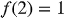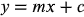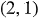1
123
views
5b
Problem

Problem 5b

Chapter 1: Functions and ModelsTextbook ExpertVerified Tutor
18 Oct 2021

Given information

The given is:

Linear function condition is.

Step-by-step explanation

Step 1.

Explanation:

The general equation of a linear function is,It satisfies the condition as,The point is.